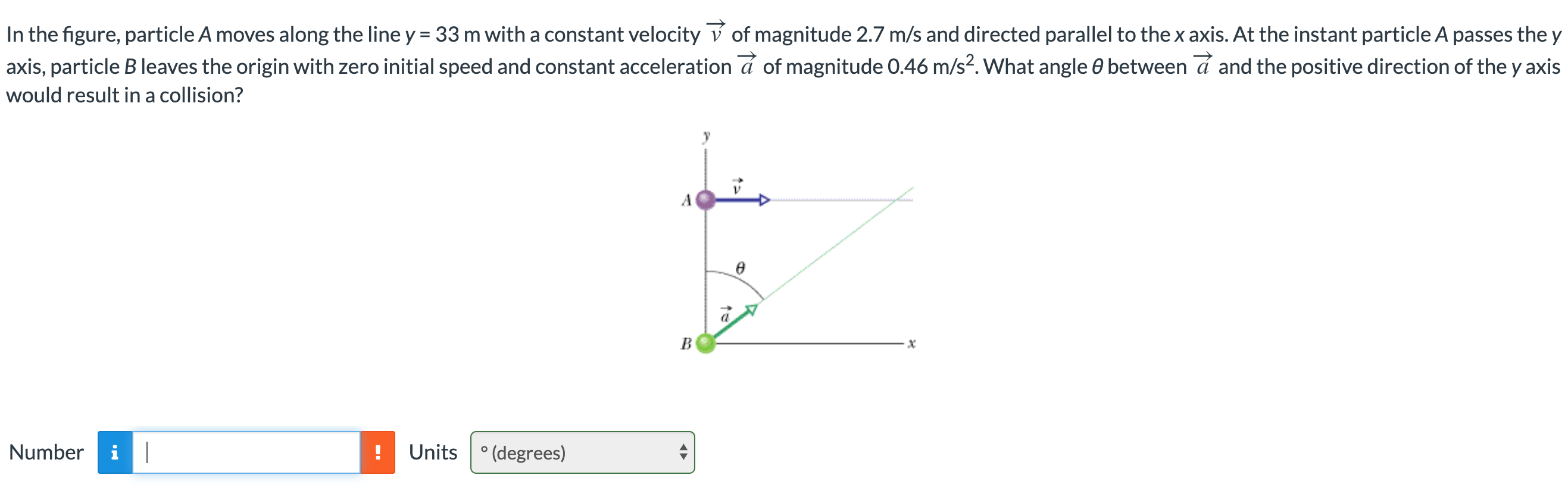Home / Answered Questions / Other / in-the-figure-particle-a-moves-along-the-line-y-33-m-with-a-constant-velocity-v-of-magnitude-2-7-m-s-aw869

# (Solved): In The Figure, Particle A Moves Along The Line Y = 33 M With A Constant Velocity V Of Magnitude 2.7 ...In the figure, particle A moves along the line y = 33 m with a constant velocity V of magnitude 2.7 m/s and directed parallel to the x axis. At the instant particle A passes the y axis, particle B leaves the origin with zero initial speed and constant acceleration Ã£ of magnitude 0.46 m/s2. What angle between a and the positive direction of the y axis would result in a collision? B Number Units Â° (degrees)

We have an Answer from Expert